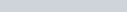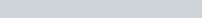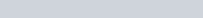# Numbers Set Analogy Questions with Solution

Numbers Set Analogy and Classification Questions with Solution in Hindi and English. New type SSC Reasoning questions, from the previous year SSC CGL, CHSL, CPO exam for the preparation and practice of upcoming exams.

## Numbers Set Analogy Reasoning Questions for SSC Exams

Select the option in which the numbers are related in the same way as are the numbers of the following set.
उस विकल्प का चयन करें, जिसमें संख्याएँ उसी प्रकार संबंधित हैं, जिस प्रकार निम्न समुच्चय (सेट) की संख्याएँ संबंधित हैं।

Q. 1: {9, 12, 18}
a) {5, 12, 24}
b) {13, 19, 31}
c) {21, 29, 44}
d) {7, 13, 27}

Ans: b) {13, 19, 31}
Solution: 12 – 9 =3 and 18-12 =6, Difference is double
Same manner in option (b)
19-13 =6 and 31-19 =12
In other option this rule is not applicable

Q.2: Select the option in which the numbers are related in the same way as are the numbers of the following set.
(42, 14, 294)
a) (30, 16, 242)
b) (34, 18, 306)
c) (21, 15, 205)
d) (36, 21, 360)

Ans : b) (34, 18, 306)
Solution:$42\times14 \div 2 = 294$
and$34 \times 18 \div 2 =306$
Others options are not following this rule.

Q.3: Select the option in which the numbers are related in the same way as are the numbers of the following set.
(4, 3, 73)
a) (7, 5, 345)
b) (5, 4, 141)
c) (6, 2, 230)
d) (5, 7, 302)

Ans : b) (5, 4, 141)
Solution: 43 + 32 =64+9 =73
53+42= 125+16 = 141

Q.4: Select the option in which the numbers share the same relationship as that shared by the given pair of numbers.
185 : 199

a) 139 : 152
b) 174 : 187
c) 126 : 134
d) 89 : 106

Ans : d) 89 : 106
Solution: 1+8+5 = 14, 185 +14 = 199
Option (d) 8+9 = 17, 89+17 = 106

Q.5: Select the option in which the numbers are related in the same way as are the numbers of the following set.
(2194, 1328, 726)

a) (1170, 570, 228)
b) (1560, 1131, 551)
c) (1406, 962, 390)
d) (1725, 997, 509)

Ans : d) (1725, 997, 509)
Solution:
133 = 2197, 2197-3 =2194,
113 = 1331, 1331-3 =1328,
93 = 729, 729-3 =726,
Same way
123=1728 , 1728-3=1725
103=1000, 1000-3 =997
83= 512, 512-3=509

Q.6: Select the option in which the numbers are related in the same way as are the numbers of the following set.
47 : 58 : 71

a) 72 : 81 : 90
b) 89 : 98 : 106
c) 76 : 89 : 105
d) 68 : 80 : 88

Ans : a) 72 : 81 : 90
Solution:
47 + (4+7) =58
58 + (5+8) = 71
Same way
72 +(7+2) = 81
81+(8+1)=90

Q.7: Select the option that is related to the third number in the same way as the second number is related to the first number.
18 : 163 : : 24 : ?

a) 216
b) 289
c) 298
d) 222

Ans : b) 289
Solution:$18^2=324, 324 \div2+1 =163$
Same way$24^2=576, 576 \div2+1 =289$

Q.8: Select the option in which the numbers share the same relationship as that shared by the given pair of numbers.
12 : 20

a) 30 : 42
b) 24 : 36
c) 42 : 57
d) 18 : 28

Ans : a) 30 : 42
Solution:
3×4 =12 : 4×5=20
Same way
5×6 =30 : 6×7=42

Q.9: Select the option in which the numbers are related in the same way as are the numbers of the following set.
(72, 56, 42)

a) (80, 48, 24)
b) (132, 110, 90)
c) (80, 68, 58)
d) (126, 102, 80)

Ans : b) (132, 110, 90)
Solution:
9×8=72, 8×7=56, 7×6=42
Same way
12×11=132, 11×10=110, 10×9=90

Q.10: Select the option in which the numbers are related in the same way as are the numbers of the following set.
(21, 13, 17)

a) (11, 21, 140)
b) (25, 35, 38)
c) (22, 57, 81)
d) (32, 46, 39)